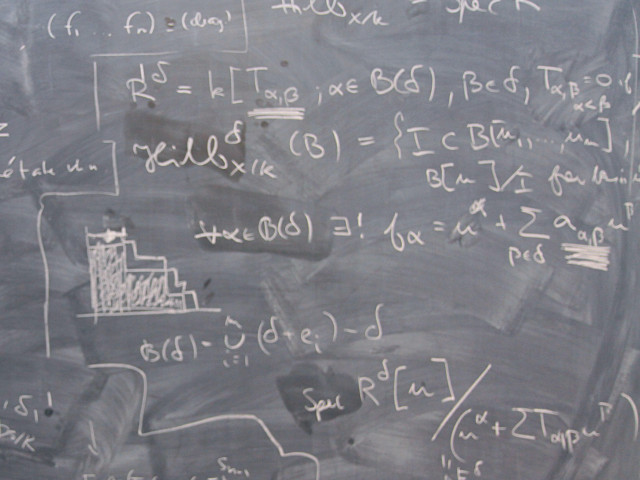# SF2525 Computational Methods for Stochastic Differential Equations and Machine Learning 7.5 creditsSF252 is a course on theory, applications and numerical methods for stochastic differential equations and its close connection to theory and computation in machine learning.

### Choose semester and course offering

Choose semester and course offering to see current information and more about the course, such as course syllabus, study period, and application information.

Headings with content from the Course syllabus SF2525 (Spring 2022–) are denoted with an asterisk ( )

## Content and learning outcomes

### Course contents

The course treats stochastic differential equations and their numerical solution, with applications in financial mathematics, machine learning, control theory and Monte Carlo methods. We discuss  basic questions for solving stochastic differential equations, e.g. to determine the price of an option is it more efficient to solve the deterministic Black and Scholes partial differential equation or use a Monte Carlo method based on stochastics.

The course treats basic theory of stochastic differential equations including weak and strong approximation, efficient numerical methods and error estimates, the relation between stochastic differential equations and partial differential equations, stochastic partial differential equations, variance reduction.

### Intended learning outcomes

After completing this PhD level course the students will be able to model, analyze and efficiently compute solutions to problems including random phenomena in science and engineering. The student learns the basic mathematical theory for stochastic differential equations, optimal control and machine learning and applies it to applications, including financial mathematics, machine learning and chemical reactions in cell biology.

More precisely the goal of the course means that the student can:

1. present some models in science and finance based on stochastic differential equations and evaluate methods to determine their solution,
2. derive and use the correspondence between expected values of stochastic diffusion processes and solutions to certain deterministic partial differential equations,
3. formulate, use and analyze the main numerical methods for stochastic differential equations, based on Monte Carlo stochastics and partial differential equations,
4. present some stochastic and deterministic optimal control problems in science and finance,
5. formulate, use and analyze deterministic and stochastic optimal control problems using both differential equations constrained minimization and dynamic programming, leading to the Hamilton-Jacobi-Bellman nonlinear partial differential equation,
6. derive the Black-Scholes equation for options in mathematical finance and analyze the alternatives to determine option prices numerically,
7. formulate, use and analyze the basic machine learning problem to determine a neural network that approximates given data using the stochastic gradient descent method,
8. use the machine learning program TensorFlow to  construct a neural network that approximates given data,
9. formulate zero-sum differential games,
10. determine and analyze reaction rate problems for stochastic differential equation with small noise using optimal control theory.

### Course disposition

No information inserted

## Literature and preparations

### Specific prerequisites

English B / English 6

The prerequisite for the course is linear algebra, calculus, differential equations, probability, programming and numerics corresponding to the first three years at KTH.

### Recommended prerequisites

No information inserted

### Equipment

No information inserted

### Literature

No information inserted

## Examination and completion

If the course is discontinued, students may request to be examined during the following two academic years.

P, F

### Examination

• LAB1 - Laboratory work, 3.5 credits, grading scale: P, F
• TEN1 - Written exam, 4.0 credits, grading scale: P, F

Based on recommendation from KTH’s coordinator for disabilities, the examiner will decide how to adapt an examination for students with documented disability.

The examiner may apply another examination format when re-examining individual students.

The examiner decides, in consultation with KTHs Coordinator of students with disabilities (Funka), about any customized examination for students with documented,lastingdisability. The examiner may allow another form of examination for reexamination of individual students.

### Other requirements for final grade

The course includes lectures, homework problems, programming using TensorFlow and student presentations of their computations inspired by recent research papers.
The homework and projects give credit points for a final written exam.

### Opportunity to complete the requirements via supplementary examination

No information inserted

### Opportunity to raise an approved grade via renewed examination

No information inserted

### ExaminerAnders Szepessy

### Ethical approach

• All members of a group are responsible for the group's work.
• In any assessment, every student shall honestly disclose any help received and sources used.
• In an oral assessment, every student shall be able to present and answer questions about the entire assignment and solution.

## Further information

### Course web

Further information about the course can be found on the Course web at the link below. Information on the Course web will later be moved to this site.

Course web SF2525

Mathematics

Second cycle